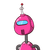# The radius and height of the cylinder are in the ratio of 5:14. Find the total surface area of the cylinder if its volume is 8800

The radius and height of the cylinder are in the ratio of 5:14. Find the total surface area of the cylinder if its volume is 8800 m3.

### 1 thought on “The radius and height of the cylinder are in the ratio of 5:14. Find the total surface area of the cylinder if its volume is 8800”

1.volume of cylinder= 8800m^

πr^h = 8800

22/7 × 5x × 5x × 14x = 8800

after calculation we find that

x = 2

so after putting value of x

and height = 28m

then, total surface area of cylinder

=2πr(r+h)

= 2×22/7×10 (10+28)

= 2388.57m^

hope u understand it

If you understand it don’t forget to rate this question